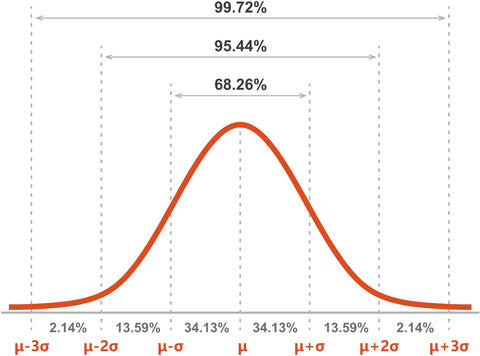# How To Calculate Standard Deviation## Standard deviation is a measure of the spread of a dataset, defined as the square root of its variance. To calculate the standard deviation of a dataset, you can use the following steps:

1. Calculate the mean (average) of the dataset.

2. For each data point, calculate the difference between the value and the mean (subtract the mean from each data point).

3. Square each difference (multiply each difference by itself).

4. Add all the squared differences together.

5. Divide the sum of the squared differences by the number of data points in the dataset (this is the variance).

6. Take the square root of the variance. This is the standard deviation of the dataset.

It is also possible to use software or calculator to do this calculation, such as excel or calculator that have the function of standard deviation.

Example: Consider a dataset {1, 2, 3, 4, 5}.

1. Mean = (1+2+3+4+5)/5 = 15/5 = 3
2. Difference = {-2,-1,0,1,2}
3. Square of difference = {4,1,0,1,4}
4. Sum of square of difference = 4+1+0+1+4 = 10
5. Variance = 10/5 = 2
6. Standard deviation = sqrt(2) = 1.41421356237